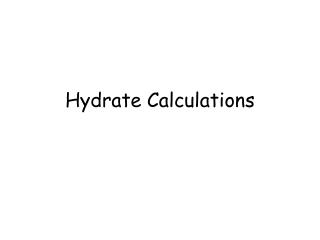DownloadDownload PresentationHydrate Calculations

# Hydrate Calculations

Download Presentation## Hydrate Calculations

- - - - - - - - - - - - - - - - - - - - - - - - - - - E N D - - - - - - - - - - - - - - - - - - - - - - - - - - -
##### Presentation Transcript

1. Hydrate Calculations

2. Hydrates • Hydrates are ionic compounds that have crystalline structures involving H2O • In MgSO4. 7H2O, there are 7 water molecules present for every 1 MgSO4. • Scientists do not always know how many H2O molecules are present, but this can be determined through calculations

3. Anhydrous Compounds • Anhydrous Compounds do not have water molecules incorporated • Instead they have the ability to absorb water from the local environment.

4. Applications • Hydrates can be used in Fire Retardation • Anhydrates can be used as dessicants (ie. Keep the atmosphere dry) • Anhydrates can indicate the presence of water.

5. Example 1: Calculate the percentage of water in Na2S2O3. 5H2O Mass of water Mass of hydrate %H2O = x 100 = x 100 = 36.30 % 5(18.02 g/mol) 248.22 g/mol

6. Example 2: Calculate the mass of water in 215 g ofNa2S2O3. 5H2O Solution 1: Use the percentage 36.30% (from the previous example). In Na2S2O3. 5H2O, there is 36.30% of water. In 215 g, there is x 215 g = 78.0 g of water 36.30 100

7. Solution 2: = = m(H2O) = 78.0 g M(H2O) M (Total) m(H2O) M(Total) m(H2O) 215 g 5(18.02 g/mol) 248.22 g/mol

8. Example 3: A hydrate of barium chloride (BaCl2.x H2O) has a mass of 1.500g. When heated to drive off the water, the residue (BaCl2) has a mass of 1.279 g Step 1: Calculate the percentage of water in the hydrate. BaCl2.x H2O (s)  BaCl2 (s) + x H2O 1.500 g 1.279g 1.500g – 1.279g = 0.221 g % H2O = x 100 = x 100 = 14.7% Mass of water Mass of hydrate 0.221 g 1.500 g

9. Step 2: Calculate the formula of the hydrate Mole Calculation: Using n=m/M BaCl2 H2O n = n= = 0.006140 mol = 0.0123 mol 1.279g 208.3 g/mol 0.221 g 18.02 g/mol

10. Step 3: Ratio Calculation: Divide by the smallest number of moles to figure out the ratio between the atoms BaCl2 H2O = = =1 = 2 Therefore the formula is BaCl2 . 2H2O 0.006140 mol 0.006140 mol 0.0123 mol 0.006140 mol

11. Example 4: A hydrate of barium hydroxide (Ba(OH)2.x H2O) has a mass of 50.0g. When heated to drive off the water, the residue (Ba(OH)2) has a mass of 27.2 g Step 1: Calculate the percentage of water in the hydrate. Ba(OH)2.x H2O (s)  Ba(OH)2 (s) + x H2O 50.0 g 27.2g 50.0g – 27.2g = 22.8 g % H2O = x 100 = x 100 = 45.6% Mass of water Mass of hydrate 22.8 g 50.0 g

12. Step 2: Calculate the formula of the hydrate Mole Calculation: Using n=m/M Ba(OH)2 H2O n = n= = 0.159 mol = 1.27 mol 27.2g 171.3 g/mol 22.8 g 18.02 g/mol

13. Step 3: Ratio Calculation: Divide by the smallest number of moles to figure out the ratio between the atoms Ba(OH)2 H2O = = =1 = 8 Therefore the formula is Ba(OH)2 . 8H2O 0.159 mol 0.159 mol 1.27 mol 0.159 mol